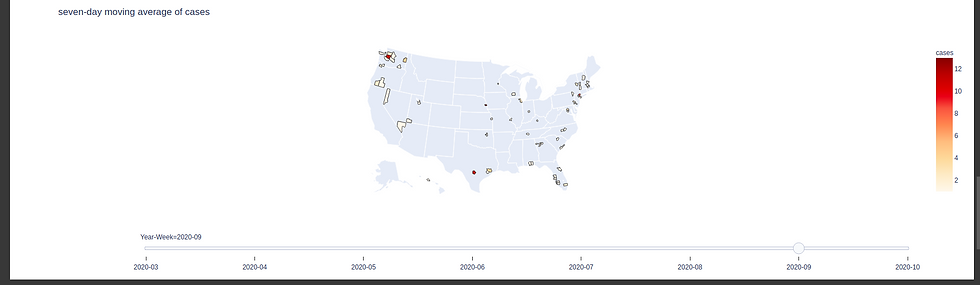top of page
Search

# Data Visualization Using Plotly | Choropleth COVID-19 ProjectRequirement:

• Successfully process data including additional columns, calculations, transformations

• Produce animated plot of case moving average

• Produce static plot of cumulative cases per 100,000

• Code does not crash

### Objectives:

• Create an animated choropleth plot using plotly that analyzes a seven-day moving average of cases for some geographic unit and sub-unit (e.g. USA and states)

• Create a second, non-animated, choropleth plot that shows cumulative cases per 100,000 people for the most recent date in the data file.

Requirements:

• Find appropriate data source that includes new COVID-19 cases per day for the geographic region. (Direct link not downloaded file.)

• Find a data source that estimates the population for the geographic region. (Direct link not downloaded file)

• Load both to a pandas dataframe

• Calculate cumulative cases per 100,000 population for the sub-region (i.e., state)

• Calculate 7-day moving average if new cases

• Plot 7-day moving average of cases on Plotly plot and animate by day (older dates on left of slider)

• Create a separate plot of cumulative cases per 100,000 population. This should be for the maximum date in the dataframe and should not be animated.

• Plots will include relevant title and hover text.

• Colors will be continous scale of your choice.

### Install the libraries

`!pip install -U plotly`

`!git clone https://github.com/nytimes/covid-19-data.git`

```import pandas as pd
import plotly.express as px

#Visualizations on US Map
df_us['new_date'] = pd.to_datetime(df_us['date'])
df_us['Year-Week'] = df_us['new_date'].dt.strftime('%Y-%U')

Output:`us100k = df_us`

Shape of dataset

```df_us = df_us.iloc[:1000,:]
df_us.shape```

Output:

`(1000, 8)`

Short And Group By

```df_us = df_us.sort_values(by=['county', 'state', 'new_date'])
df_us_week = df_us.groupby(['county', 'state', 'fips', 'Year-Week']).first().reset_index()
df_us_week

OutputCount the cases

`df_us_week['cases'].max(), df_us_week['cases'].min()`

Output

`(91, 1)`

```from urllib.request import urlopen
import json
with urlopen('https://raw.githubusercontent.com/plotly/datasets/master/geojson-counties-fips.json') as response:

counties["features"]```

Output:

```{'geometry': {'coordinates': [[[-113.931799, 42.535275],
[-113.932904, 42.765032],
[-113.763862, 42.764508],
[-113.713928, 42.849733],
[-113.714701, 43.20003],
[-113.413693, 43.199785],
[-113.413026, 42.84925],
[-113.472155, 42.849218],
[-113.472177, 42.669251],
[-113.557609, 42.656416],
[-113.655338, 42.535663],
[-113.779811, 42.55687],
[-113.931799, 42.535275]]],
'type': 'Polygon'},
'id': '16067',
'properties': {'CENSUSAREA': 757.591,
'COUNTY': '067',
'GEO_ID': '0500000US16067',
'NAME': 'Minidoka',
'STATE': '16'},
'type': 'Feature'}```

Create an animated choropleth plot using plotly that analyzes a seven-day moving average of cases for some geographic unit and sub-unit (e.g. USA and states)

```df_us_week = df_us_week.sort_values(by=['Year-Week'])
fig = px.choropleth(df_us_week, geojson=counties, locations='fips', color='cases',
color_continuous_scale=px.colors.sequential.OrRd,
title = "seven-day moving average of cases",
scope="usa",
animation_frame="Year-Week",
)
fig.show()

```

Output:Create a second, non-animated, choropleth plot that shows cumulative cases per 100,000 people for the most recent date in the data file.

```us100k=us100k.loc[us100k['cases'] <= 100000]
fig = px.choropleth(us100k, geojson=counties, locations='fips', color='cases',
color_continuous_scale=px.colors.sequential.OrRd,
title = "cumulative cases per 100,000 people",
scope="usa",

)